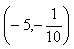Holes
Example 1

Find the points of discontinuity for the rational function.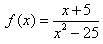Step 1. Factor the rational function completely.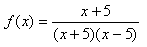Step 2. Determine the points of discontinuity by setting the factors in the denominator equal to zero and solving.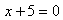or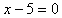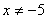or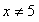Step 3. Simplify the rational function.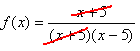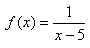Step 4. Determine the vertical asymptote.

x = 5 is a vertical asymptote because it is a zero of the denominator only.

Step 5. Determine the coordinates of the hole.

There is a hole at x = -5 because the factor (x + 5) was cancelled in both the numerator and denominator. To determine the y-value, plug x = -5 into the simplified function and solve.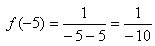Coordinates of the hole: